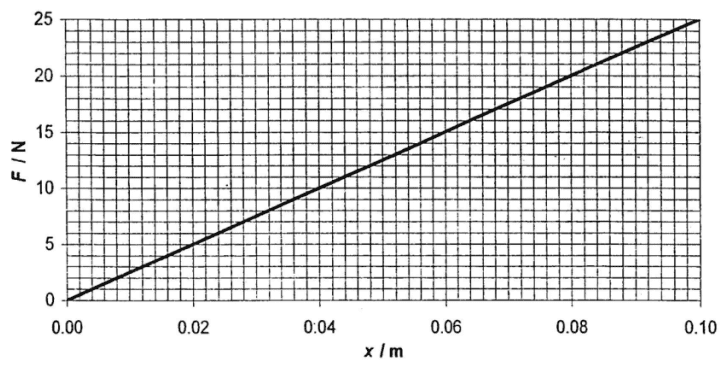# Additional energy stored in spring

## Homework Statement

The graph shows the variation with extension x of the load F on a certain springA load of 10 N is placed on the spring. How much additional elastic potential energy will be stored in the spring if it is then extended a further 0.040 m?
a. 0.200 J
b. 0.450 J
c. 0.600 J
d. 0.800 J

F = k x
E = 1/2 k x2

## The Attempt at a Solution

Load of 10 N means the extension is 0.04 m and extended further 0.04 m means that the extension is 0.08 m, right?

I got (c) but the answer key is (a) ??

Thanks

PeroK
Homework Helper
Gold Member
2020 Award

## Homework Statement

The graph shows the variation with extension x of the load F on a certain spring
View attachment 237172
A load of 10 N is placed on the spring. How much additional elastic potential energy will be stored in the spring if it is then extended a further 0.040 m?
a. 0.200 J
b. 0.450 J
c. 0.600 J
d. 0.800 J

F = k x
E = 1/2 k x2

## The Attempt at a Solution

Load of 10 N means the extension is 0.04 m and extended further 0.04 m means that the extension is 0.08 m, right?

I got (c) but the answer key is (a) ??

Thanks

I think you are correct.

Thank you